Math resources Geometry

3D shapes

Triangular pyramid

# Triangular pyramid

Here you will learn about triangular pyramids, including what a triangular pyramid is and how to find the volume and surface area of triangular pyramids.

Students will first learn about triangular pyramids as part of geometry in 1 st grade. In middle school and high school, students will expand their knowledge of triangular pyramids when they learn to find the volume and the surface area of a triangular pyramid.

## What is a triangular pyramid?

A triangular pyramid is a three-dimensional shape with 4 flat triangular faces, 6 edges and 4 vertices. Another name for a triangular pyramid is tetrahedron.

\bf{4} faces \bf{6} edges \bf{4} vertices

### Types of triangular pyramids

There are two main types of triangular pyramids, right triangular pyramids and irregular pyramids.

A right triangular pyramid is a triangular pyramid which has an equilateral triangle as its base and the apex is directly above the center of the triangle base, forming a right angle. The other 3 faces are congruent isosceles triangles. If all of the faces are congruent equilateral triangles it is a regular triangular pyramid or regular tetrahedron.

An irregular triangular pyramid is a triangular pyramid where its base is not an equilateral triangle.

### Net of a triangular pyramid

The net of a triangular pyramid is made of 4 triangles. This net shows 4 equilateral triangles, therefore, this is the net of a regular tetrahedron. The prefix “tetra” comes from the 4 triangular faces.

### Volume of a triangular pyramid

The volume of a triangular pyramid can be found by using the formula,

\text{Volume}=\cfrac{1}{3}\times \text{area of base} \times \text{height}.

You may be given the area of the triangular base or be asked to work it out. The pyramid height should be perpendicular to its base.

This can be written as,

V=\cfrac{1}{3} \, Bh

where,

• V represents the volume of the pyramid.
• B represents the area of the base of the pyramid.
• h represents the perpendicular height of the pyramid.

### Surface area of a triangular pyramid

The surface area of a triangular pyramid is calculated by finding the area of each of
the triangular faces and adding them together.

If the faces of the triangular pyramid are all the same size, you can find the area of one face and multiply it by 4.

If the faces are different, you will need to work out the area of each face and add them together.

For example, find the surface area of the regular tetrahedron.

Area of each triangular face = 6 \mathrm{~cm}^2

There are 4 triangular faces, so multiply 6 \mathrm{~cm}^2 by 4 to give 24 \mathrm{~cm}^2.

Total surface area = 24 \mathrm{~cm}^2

### What is a triangular pyramid?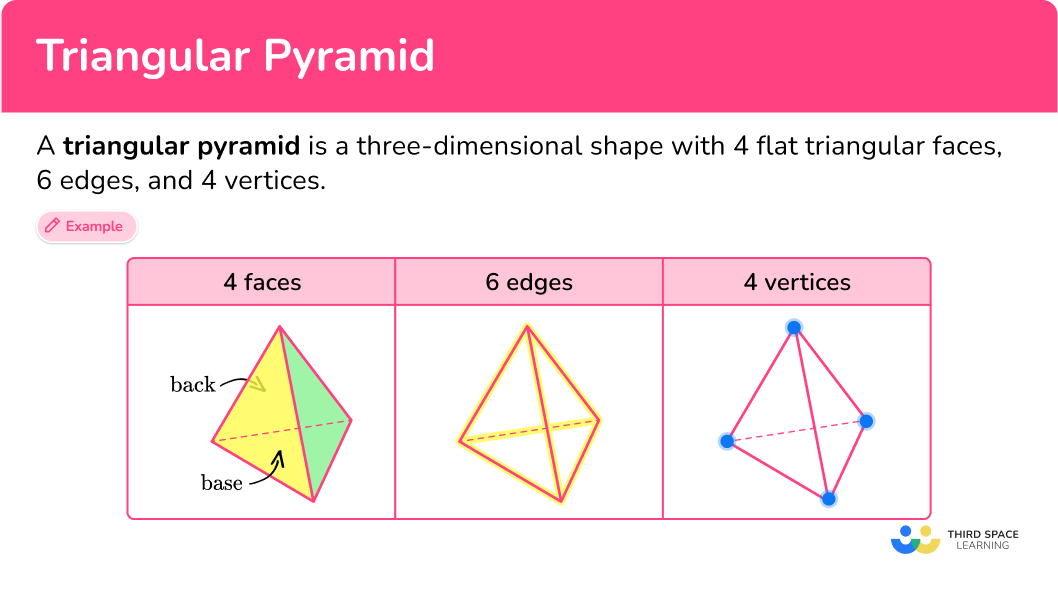## Common Core State Standards

Compose two-dimensional shapes (rectangles, squares, trapezoids, triangles, half-circles, and quarter-circles) or three-dimensional shapes (cubes, right rectangular prisms, right circular cones, and right circular cylinders) to create a composite shape, and compose new shapes from the composite shape.

Represent three-dimensional figures using nets made up of rectangles and triangles, and use the nets to find the surface area of these figures. Apply these techniques in the context of solving real-world and mathematical problems.

Solve real-world and mathematical problems involving area, volume and surface area of two- and three-dimensional objects composed of triangles, quadrilaterals, polygons, cubes, and right prisms.

## How to identify a triangular pyramid

In order to identify a triangular pyramid, you will:

1. Look for the characteristics of a triangular pyramid.
2. State whether or not the shape is a triangular pyramid.
3. If the shape is not a triangular pyramid, explain what characteristics are different.

## Triangular pyramid examples

### Example 1: identifying triangular pyramids

Look at the image below and determine if it’s a triangular pyramid or not.

1. Look for the characteristics of a triangular pyramid.

A triangular pyramid is a 3D shape with 4 triangular faces, 6 edges and 4 vertices.

This shape has 2 triangular faces, 3 rectangular faces, 9 edges and 6 vertices.

2State whether or not the shape is a triangular pyramid.

This shape is not a triangular pyramid, it is a triangular prism.

3If the shape is not a triangular pyramid, explain what characteristics are different.

The triangular prism has 2 triangular side faces and 3 rectangular faces, 9 edges and 6 vertices.

### Example 2: identifying triangular pyramids

Look at the image below and determine if it’s a triangular pyramid or not.

A triangular pyramid is a 3D shape with 4 triangular faces, 6 edges and 4 vertices.

This shape has 4 triangular faces, 6 edges and 4 vertices.

This shape is a triangular pyramid.

## How to calculate the volume of a triangular pyramid

In order to calculate the volume of a triangular pyramid:

1. Calculate the area of the base.
2. Substitute values into the formula and solve.
3. Write the answer, including the units.

### Example 3: calculating the volume

Find the volume of the pyramid. The area of the base is 20 \mathrm{~cm}^2.

The area of the base is given to you and is 20 \mathrm{~cm}^2.

The volume of the triangular pyramid can be found by using the formula,

V=\cfrac{1}{3} \, Bh.

Where,

\begin{aligned}& B=20 \mathrm{~cm}^2 \text { and } \\\\ & h=6 \mathrm{~cm}\end{aligned}

Substitute the values that have been given into the formula:

V=\cfrac{1}{3}\times 20 \times 6.

\text {Volume}=\cfrac{1}{3}\times 20 \times 6 =40.

The volume of the triangular pyramid is 40 \mathrm{~cm}^3.

### Example 4: calculating height given the volume and area

Find the height of the pyramid with volume 150 \mathrm{~cm}^3 and the base area of 60 \mathrm{~cm}^2.

The area of the base is given to you and is 60 \mathrm{~cm}^2.

The volume of a triangular pyramid can be found by using the formula,

V=\cfrac{1}{3} \, Bh.

Where,

B=60 \mathrm{~cm}^2 , and h is unknown.

Substitute the values that have been given into the formula,

150=\cfrac{1}{3}\times 60 \times h.

This can be rearranged to give,

h=\cfrac{150\times 3}{60}=7.5.

The height of the pyramid is 7.5 \mathrm{~cm}.

### Example 5: calculating base area given the height of the pyramid

The volume of the pyramid below is 40 \mathrm{~m}^3. Find the base area.

The volume of a triangular pyramid can be found by using the formula,

V=\cfrac{1}{3} \, Bh.

Substitute the values that have been given into the formula,

40=\cfrac{1}{3}\times B\times 10.

This can be rearranged to give,

B=\cfrac{40\times 3}{10}=12.

The area of the base of the pyramid is 12 \mathrm{~cm}^2.

## How to calculate the surface area of a triangular pyramid

In order to find the surface area of a triangular pyramid:

1. Calculate the area of each face.
2. Add the area of each face together.
3. Include the units.

### Example 6: working out the total surface area

Find the total surface area of a triangular pyramid made of equilateral triangles. The area of each face is 20 \mathrm{~cm}^2.

This step is not needed, because you are given the area of each face, which is 20 \mathrm{~cm}^2.

A triangular pyramid is made of 4 triangular faces.

You are told that all 4 faces are the same and each face has an area of 20 \mathrm{~cm}^2.

Therefore, the total surface area can be found by multiplying 20 by 4.

4\times 20=80

The total surface area is 80 \mathrm{~cm}^2.

### Teaching tips for triangular pyramid

• Provide students with manipulatives, such as pyramid-shaped blocks or cut-outs, that they can hold and manipulate. Encourage them to examine the different faces, edges, and vertices of the pyramid.

• Help students make connections between triangular pyramids and other geometric shapes. For example, discuss how a triangular pyramid can be seen as a combination of a triangle and a point (vertex), or how it relates to the concept of a tetrahedron. This helps deepen their understanding of geometric relationships.

• Provide students with problem-solving activities that involve triangular pyramids, instead of practice worksheets. For example, give them a scenario where they need to calculate the height of a pyramid or find the dimensions of a base given the volume.

### Easy mistakes to make

• Not using the perpendicular height
The height of the pyramid needs to be the perpendicular height. This is the height that is at a right-angle to the base.

• Using the wrong units
Remember to use cubic units for volume such as \mathrm{cm}^3 \text { or } \mathrm{m}^3. Square units are for area, for example ​​ \mathrm{cm}^2 or \mathrm{m}^2.

• Mixing up the different triangular pyramid formulas
There are many different volume formulas in math. Make sure that you use the correct one to work out the volume of a pyramid.
\text{Volume}=\cfrac{1}{3}\times \text{area of base} \times \text{height}
Or
V=\cfrac{1}{3} \, Bh.

### Practice triangular pyramid questions

1. Which shape is a triangular pyramid?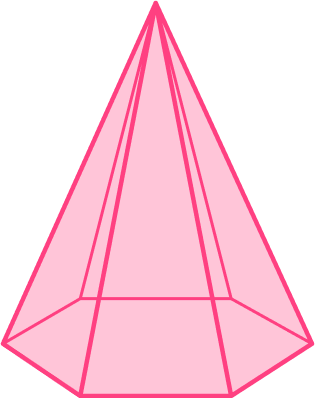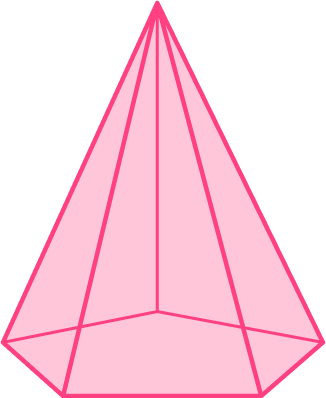A triangular pyramid is a 3D shape with 4 triangular faces, 6 edges and 4 vertices.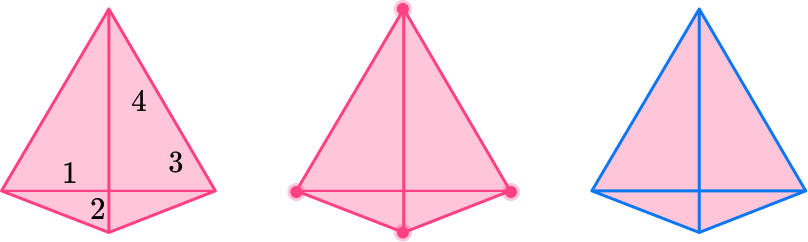2. Which shape is a triangular pyramid?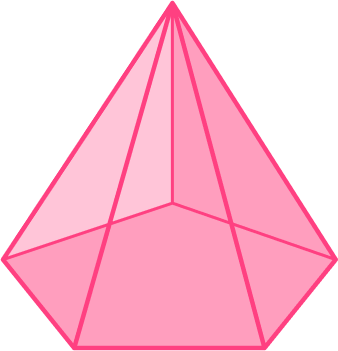A triangular pyramid is a 3D shape with 4 triangular faces, 6 edges and 4 vertices.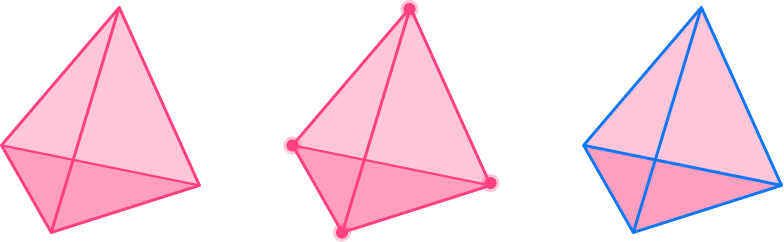3. The area of the base of this triangular pyramid is 40 \mathrm{~cm}^2. Find its volume.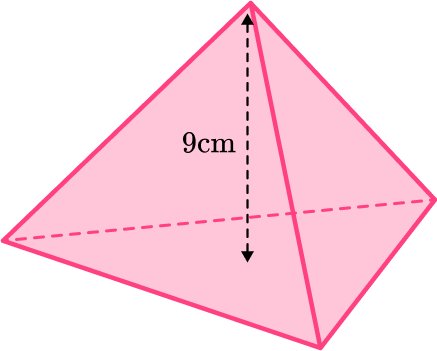360 \mathrm{~cm}^2120 \mathrm{~cm}^2120 \mathrm{~cm}^3360 \mathrm{~cm}^3You can find the volume of a pyramid by using the formula:

V=\cfrac{1}{3} \, Bh.

Where,

B=40 \mathrm{~cm}^2 \text { and } h=9 \mathrm{~cm}.

Substitute the values that have been given into the formula and work out the volume,

V=\cfrac{1}{3}\times 40 \times 9=120.

The volume of the triangular pyramid is 120 \mathrm{~cm}^3.

4. The volume of a triangular pyramid is 100 \mathrm{~cm}^3. The area of its base is 25 \mathrm{~cm}^2. Find the height of the triangular pyramid.

24 \mathrm{~cm}12 \mathrm{~cm}4 \mathrm{~cm}40 \mathrm{~cm}Use the formula for volume of a pyramid:

V=\cfrac{1}{3} \, Bh.

Where,

V=100 \mathrm{~cm}^3 \text { and } B=25 \mathrm{~cm}^2.

Substitute the values that have been given into the formula,

100=\cfrac{1}{3}\times 25 \times h.

You can rearrange this to find the height,

h=\cfrac{100\times 3}{25}=12.

The height of the triangular pyramid is 12 \mathrm{~cm}.

5. The volume of a triangular pyramid is 80 \mathrm{~cm}^3. The height is 9.7 \mathrm{~cm}. Find the area of the base of the triangular pyramid. Round your answer to the tenths place.

24.8 \mathrm{~cm}^28.2 \mathrm{~cm}^268.0 \mathrm{~cm}^224.7 \mathrm{~cm}^2Use the formula for volume of a pyramid,

V=\cfrac{1}{3} \, Bh.

Where,

V=80 \mathrm{~cm}^3 \text { and } h=9.7 \mathrm{~cm}.

Substitute the values that have been given into the formula,

80=\cfrac{1}{3}\times B \times 9.7

You can rearrange this to find the base area,

B=\cfrac{80\times 3}{9.7}=24.742…

The area of the base is 24.7 \mathrm{~cm}^2 when rounded to the tenths place.

6. A triangular pyramid is made from 4 equilateral triangles. If the area of one of the faces is 21 \mathrm{~cm}^2, find the total surface area of the triangular pyramid.

61 \mathrm{~cm}^384 \mathrm{~cm}^384 \mathrm{~cm}^261 \mathrm{~cm}^2A triangular pyramid has 4 faces. If all the faces are the same, you can find the total surface area by multiplying by 4.

You are told that the pyramid is made up of 4 equilateral triangles with an area of 21 \mathrm{~cm}^2.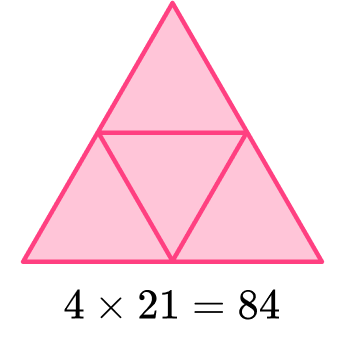The total surface area is 84 \mathrm{~cm}^2.

## Triangular pyramid FAQs

What is a triangular pyramid?

A triangular pyramid, also known as a tetrahedron, is a polyhedron with a triangular base and three triangular faces that converge at a single point called the vertex.

What is a pyramid?

A pyramid is a polyhedra with a flat base and triangular sides which meet at the apex. The flat base can be any polygon. Pyramids can come in different forms such as a square pyramid, a rectangular pyramid or even a pentagonal pyramid.

How can I find the volume of a regular triangular pyramid?

To calculate the volume of a triangular pyramid, you need to multiply the base area by the height and divide the result by 3. The formula is:

\text { Volume }=\cfrac{1}{3} \times \text { Base Area } \times \text { Height }

The base area is the area of the triangular base, and the height is the perpendicular distance from the base to the apex.

How can I find the surface area of a regular triangular pyramid?

To calculate the surface area of a triangular pyramid, you need to find the area of each triangular face and sum them up. You can use the surface area of a triangular pyramid formula:

\text { Surface Area }=\cfrac{1}{2} \times \text { Base Perimeter } \times \text { Slant Height }+ \text { Base Area }

The base perimeter is the sum of the lengths of the base triangle’s sides, and the slant height is the distance from the apex to the base along one of the triangular faces.

## Still stuck?

At Third Space Learning, we specialize in helping teachers and school leaders to provide personalized math support for more of their students through high-quality, online one-on-one math tutoring delivered by subject experts.

Each week, our tutors support thousands of students who are at risk of not meeting their grade-level expectations, and help accelerate their progress and boost their confidence.